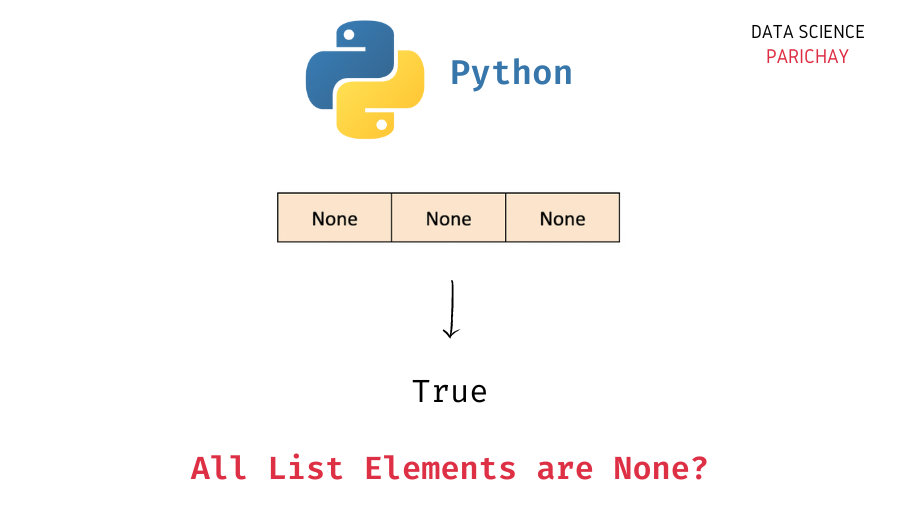# Python – Check If All Elements in List are None

In this tutorial, we will look at how to check if all the elements in a list are None (equal to the value `None`) or not in Python with the help of some examples.

## How to check if all the list items are `None`?

You can use the Python built-in `all()` function to check if all the elements in a list are None or not by comparing each value in the list with `None`.

The `all()` function takes in an iterable as an argument and returns `True` if all the values in the iterable are truthy (represent `True` in a boolean context).

So, to check if all the values in a given list are zero or not, use the `all()` function to check if all the values are equal `None`. The following is the syntax –

```# check if all the list values are None
all(val == None for val in ls)```

It returns `True` if all the values in the list are equal to `None`.

Note that there are other methods as well that you can use to check if all list values are None or not. Some of them are –

• Iterate through the list and keep a count of values that are `None`. If this count is the same as the length of the list, you can say that all values are None.
• Create a set from list values and check if the set is equal to `{None}`.

## Examples

Let’s now look at some examples of using the above methods. First, we will create a few lists that we’ll use to demonstrate the methods.

```# list with all values as zero
ls1 = [None, None, None, None]
# list with different values
ls2 = [0, 1, 2, None, 4, 5, 5]
# empty list
ls3 = []

# display the lists
print(ls1)
print(ls2)
print(ls3)```

Output:

📚 Data Science Programs By Skill Level

Introductory

Intermediate ⭐⭐⭐

🔎 Find Data Science Programs 👨‍💻 111,889 already enrolled

Disclaimer: Data Science Parichay is reader supported. When you purchase a course through a link on this site, we may earn a small commission at no additional cost to you. Earned commissions help support this website and its team of writers.

```[None, None, None, None]
[0, 1, 2, None, 4, 5, 5]
[]```

Here, we created three lists – `ls1`, `ls2`, and `ls3`. The list `ls1` contains only the value `None` as its elements. The list `ls2` has repeated values but not all values are equal to `None` and the list `ls3` is empty (it does not contain any elements).

### Example 1 – Check if all the list elements are `None` using `all()`

The idea here is to use the `all()` function to check if each list element is equal to `None`.

You can use a list comprehension to create a list of boolean values – whether a list element is equal to `None` or not and then pass this resulting list as an argument to the `all()` function.

Let’s apply this to the lists `ls1` and `ls2` created above.

```# check if all list values are None
print(all([val == None for val in ls1]))
print(all([val == None for val in ls2]))```

Output:

```True
False```

We get `True` for `ls1` and `False` for `ls2`.

If you apply this method to an empty list, you’ll get `True` as the output.

`all([val == None for val in ls3])`

Output:

`True`

Note that the `all()` function takes an iterable as an argument, so you can directly use an iterator (without using a list comprehension).

```# check if all list values are None
print(all(val == None for val in ls1))
print(all(val == None for val in ls2))
print(all(val == None for val in ls3))```

Output:

```True
False
True```

We get the same results as above.

### Example 2 – Check if all list elements are `None` using a for loop

The idea, here, is to iterate through the list and keep a count of values that are equal to `None`. If the resulting count is the same as the length of the list, we can say that all the values in the list are None.

```def all_list_elements_none(ls):
count = 0
for val in ls:
if val == None:
count += 1
return count == len(ls)

# check if all list values are none
print(all_list_elements_none(ls1))
print(all_list_elements_none(ls2))
print(all_list_elements_none(ls3))```

Output:

```True
False
True```

We get `True` for `ls1` and `False` for `ls2`. Note that here we get `True` for an empty list.

### Example 3 – Check if all the list elements are `None` using a set

In this method, we create a set from the list elements and check if the resulting set is equal to the set `{None}`. If the list has only `None` as its elements, the resulting set will be `{None}` (that is, a set with only `None` as the value).

```# check if all list values are zero
print(set(ls1) == {0})
print(set(ls2) == {0})
print(set(ls3) == {0})```

Output:

```True
False
False```

We get `True` for `ls1` and `False` for `ls2` and `ls3`. Note that here we get `False` for an empty list.

## Summary

In this tutorial, we looked at some different methods to check if all the values in a list are None or not. The following are the different methods covered –

• Use the Python built-in `all()` function to check if each list element is equal to `None`.
• Iterate through the list elements and track the count of values that are `None` and then compare this count with the length of the list.
• Convert the list to a set and check if the resulting set is `{None}`.

You might also be interested in –

•